# Texas Go Math Grade 2 Lesson 14.5 Answer Key Take Apart Two-Dimensional Shapes

Refer to our Texas Go Math Grade 2 Answer Key Pdf to score good marks in the exams. Test yourself by practicing the problems from Texas Go Math Grade 2 Lesson 14.5 Answer Key Take Apart Two-Dimensional Shapes.

## Texas Go Math Grade 2 Lesson 14.5 Answer Key Take Apart Two-Dimensional Shapes

Explore

Circle the names of shapes you see inside the blue shape.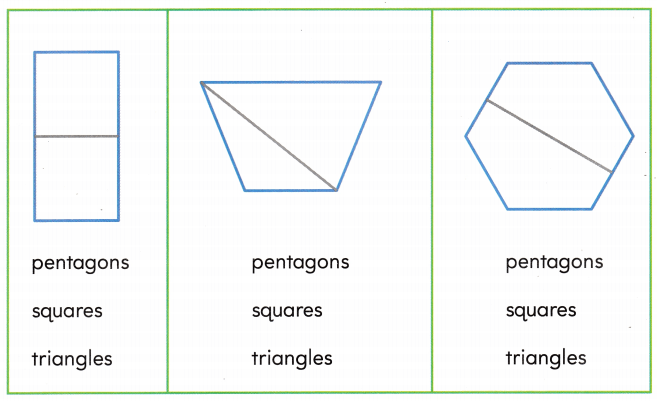FOR THE TEACHER • Have children identify the shapes they see in the quadrilaterals and the hexagon.Explanation:
I can see squares, triangles and pentagons in the blue shape.

Math Talk
Mathematical Processes

Describe the shapes using the words whole and parts.

Model and Draw

You can fold and cut this hexagon to make two quadrilaterals.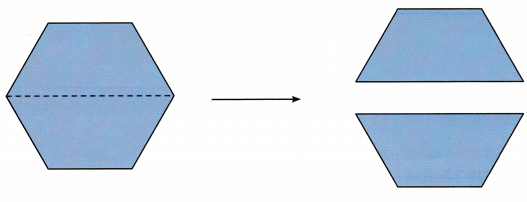If i fold and cut this hexagon it forms two quadrilaterls.

Share and Show

Use paper shapes. Fold to make two new shapes. Cut along the fold. Draw to show what you did. Name the new shapes.

hexagon
triangle
pentagon
rectangle
square

Question 1.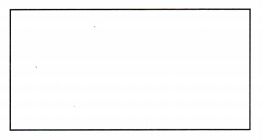Explanation:
The two new shapes are squares.

Question 2.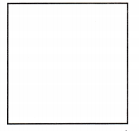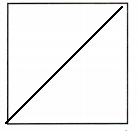Explanation:

Problem Solving

Use paper shapes. Fold to make two new shapes. Cut along the fold. Draw to show what you did. Name the new shapes.

hexagon
triangle
pentagon
rectangle
square

Question 3.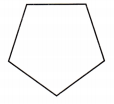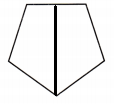Explanation:

Question 4.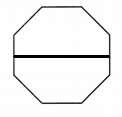Explanation:

Question 5.
H.O.T. Alberto cut this shape to make three rectangles. His three rectangles are different sizes. Draw lines to show rectangles Alberto could make.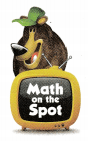Explanation:
Alberto cut this shape to make three rectangles. I drew lines to show the rectangles of different sizes that Alberto could make.

Question 6.
H.O.T. Multi-Step Monica makes new shapes that are all the same size. She starts to draw lines to show what she did. Complete Monica’s drawing. How many shapes did she make?Name the new shapes. _____________Explanation:
The new shapes Monica made are triangles. all the triangles are of equal size.

Question 7.
Maria folds this shape to make new shapes. What new shapes did she make?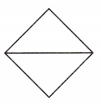(A) triangles
(B) squares
(C) pentagons
(A) triangles

Explanation:
Maria folds this shape to make new shapes. The two new shapes she get are triangles.

Question 8.
Analyze Sue folds this shape to make new shapes. What new shapes did she make?(A) hexagons
(B) pentagons

Explanation:
Sue folds this shape to make new shapes. She made two new quadrilaterals.

Question 9.
Draw a line on the shape below to show how you could fold and cut the shape to make two new shapes. Name the new shapes.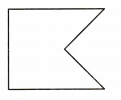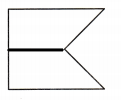Explanation:
I drew a line on the shape above to show how i could fold and cut the shape to make two new shapes. The shapes are quadrilaterals.

Question 10.
Texas Test Prep Which shows a way to fold and cut a rectangle to make two triangles?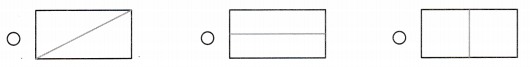Explanation:
I marked the rectangle that makes the two triangles when it is folded.

TAKE HOME ACTIVITY • Have your child explain how he or she solved one of the problems in this lesson.

### Texas Go Math Grade 2 Lesson 14.5 Homework and Practice Answer Key

Use paper shapes. Fold to make two new shapes. Cut along the fold. Draw to show what you did. Name the new shapes.

Question 1.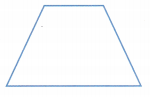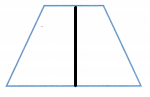Explanation:

Question 2.Explanation:

Problem Solving

Question 3.
Multi-Step Emma makes new shapes that are all the same size She starts to draw lines to show what she did. Complete Emma’s drawing.
How many shapes did she make?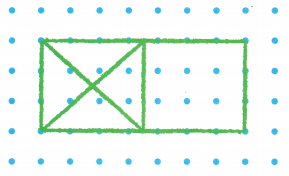Name the new shapes. ______________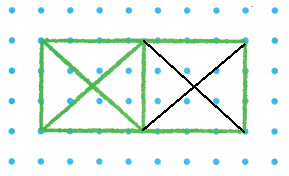Explanation:
The new shapes that formed are triangles.

Lesson Check

Question 4.
Which shows a way to fold and cut a rectangle to make two triangles?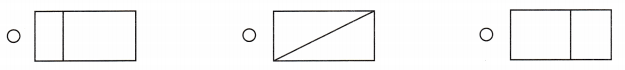Explanation:
I marked that rectangle which makes two triangles when folded and cut.

Question 5.
Willie folds this shape to make new shapes. What new shapes did he make?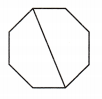(A) pentagons
(B) triangles
(A) pentagons

Explanation:
Willie folds this shape to make new shapes. She can make 2 pentagons.

Question 6.
Amy folds this shape to make new shapes. What new shapes did she make?(A) triangles
(B) squares

Explanation:
Amy folds this shape to make new shapes. The new shapes are quadrilaterals.

Question 7.
Gavin wants to plant three kinds of flowers in his garden. He separates the garden into three parts. What shapes did Gavin make?(A) squares
(B) triangles
(C) rectangles